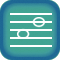not translated

Skills covered:
 Major and Minor SecondsMajor and Minor Thirds The Tritone IntervalPerfect Intervals
Новые курсы в настоящее время доступны только на английском языке . Продолжайте смотреть эту страницу , так как мы сейчас работаем над переводом на другие языки.

1.  Major and minor seconds
Lesson

2.  Aural recognition of m2, M2, P4 and P8 (melodic)
Lesson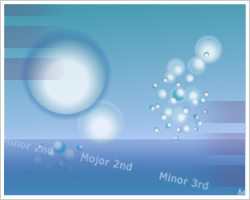Мелодические капли
Level 4

3.  Aural recognition of m2, M2, P4 and P8 (harmonic)
Lesson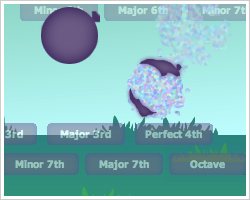Гармонические капли
Level 4

4.  M2, P4, P5 and P8 in major keys (solfege)
Lesson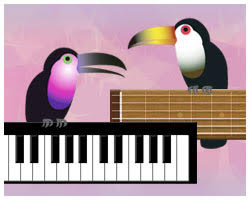Три ноты (мажор)
Level 4

5.  M2, P4, P5 and P8 in minor keys (solfege)
Lesson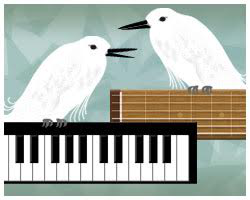Три ноты (минор)
Level 1

6.  m2, P4, P5 and P8 in major keys (solfege)
LessonТри ноты (мажор)
Level 5

7.  m2, P4, P5 and P8 in minor keys (solfege)
LessonТри ноты (минор)
Level 2

8.  Notation of M2, P4, P5 and P8
Lesson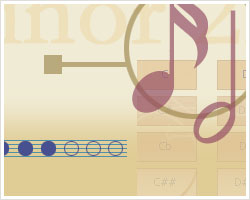Блеск нотации (интервалы)
Level 2

9.  Notation of m2, P4, P5 and P8
LessonБлеск нотации (интервалы)
Level 3

10.  Aural recognition of M2, P4, P5 and P8 (melodic)
Lesson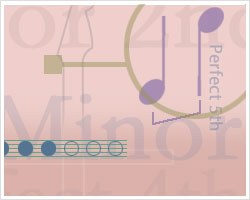Блеск интервалов (мел.)
Level 2

11.  Aural recognition of M2, P4, P5 and P8 (harmonic)
Lesson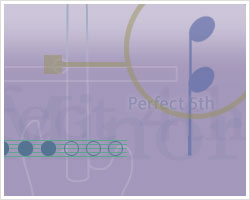Блеск интервалов (гарм.)
Level 2

12.  Aural recognition of m2, P4, P5 and P8 (melodic)
LessonБлеск интервалов (мел.)
Level 3

13.  Aural recognition of m2, P4, P5 and P8 (harmonic)
LessonБлеск интервалов (гарм.)
Level 3

14.  Aural recognition of m2, M2, P4, P5 and P8 (melodic) 1
LessonМелодические капли
Level 5

15.  Aural recognition of m2, M2, P4, P5 and P8 (harmonic) 1
LessonГармонические капли
Level 5

16.  m2, M2, P4, P5 and P8 in major keys (solfege)
LessonТри ноты (мажор)
Level 6

17.  m2, M2, P4, P5 and P8 in minor keys (solfege)
LessonТри ноты (минор)
Level 3

18.  Notation of m2, M2, P4, P5 and P8
LessonБлеск нотации (интервалы)
Level 4

19.  Aural recognition of m2, M2, P4, P5 and P8 (melodic) 2
LessonБлеск интервалов (мел.)
Level 4

20.  Aural recognition of m2, M2, P4, P5 and P8 (harmonic) 2
LessonБлеск интервалов (гарм.)
Level 4

21.  Major and minor thirds
Lesson

22.  Aural recognition of m3, M3, P5 and P8 (melodic)
LessonМелодические капли
Level 6

23.  Aural recognition of m3, M3, P5 and P8 (harmonic)
LessonГармонические капли
Level 6

24.  M3, P4, P5 and P8 in major keys (solfege)
LessonТри ноты (мажор)
Level 7

25.  m3, P4, P5 and P8 in minor keys (solfege)
LessonТри ноты (минор)
Level 4

26.  Notation of M3, P4, P5 and P8
LessonБлеск нотации (интервалы)
Level 5

27.  Notation of m3, P4, P5 and P8
LessonБлеск нотации (интервалы)
Level 6

28.  Aural recognition of M3, P4, P5 and P8 (melodic)
LessonБлеск интервалов (мел.)
Level 5

29.  Aural recognition of M3, P4, P5 and P8 (harmonic)
LessonБлеск интервалов (гарм.)
Level 5

30.  Aural recognition of m3, P4, P5 and P8 (melodic)
LessonБлеск интервалов (мел.)
Level 6

31.  Aural recognition of m3, P4, P5 and P8 (harmonic)
LessonБлеск интервалов (гарм.)
Level 6

32.  Aural recognition of m3, M3, P4, P5, and P8 (melodic) 1
LessonМелодические капли
Level 7

33.  Aural recognition of m3, M3, P4, P5, and P8 (harmonic) 1
LessonГармонические капли
Level 7

34.  Notation of m3, M3, P4, P5 and P8
LessonБлеск нотации (интервалы)
Level 7

35.  Aural recognition of m3, M3, P4, P5, and P8 (melodic) 2
LessonБлеск интервалов (мел.)
Level 7

36.  Aural recognition of m3, M3, P4, P5, and P8 (harmonic) 2
LessonБлеск интервалов (гарм.)
Level 7

37.  Aural recognition of m2, M2, m3 and M3 (melodic) 1
LessonМелодические капли
Level 8

38.  Aural recognition of m2, M2, m3 and M3 (harmonic) 1
LessonГармонические капли
Level 8

39.  m2, M2, m3 and M3 in major keys (solfege)
LessonТри ноты (мажор)
Level 8

40.  m2, M2, m3, M3 in minor keys (solfege)
LessonТри ноты (минор)
Level 5

41.  Notation of m2, M2, m3 and M3
LessonБлеск нотации (интервалы)
Level 8

42.  Aural recognition of m2, M2, m3, M3 (melodic) 2
LessonБлеск интервалов (мел.)
Level 8

43.  Aural recognition of m2, M2, m3 and M3 (harmonic) 2
LessonБлеск интервалов (гарм.)
Level 8

44.  The diminished fifth (tritone)
Lesson

45.  Aural recognition of m2, M2, m3, M3 and d5 (melodic) 1
LessonМелодические капли
Level 9

46.  Aural recognition of m2, M2, m3, M3 and d5 (harmonic) 1
LessonГармонические капли
Level 9

47.  m2, M2, m3, M3 and tritone in major keys (solfege)
LessonТри ноты (мажор)
Level 9

48.  m2, M2, m3, M3 and tritone in minor keys (solfege)
LessonТри ноты (минор)
Level 6

49.  m2, M2, m3, M3, P4 and d5 in minor keys (solfege)
LessonТри ноты (минор)
Level 7

50.  m2, M2, m3, M3, P4, d5 and P5 in minor keys (solfege)
LessonТри ноты (минор)
Level 8

51.  Notation of m2, M2, m3, M3 and tritone
LessonБлеск нотации (интервалы)
Level 9

52.  Aural recognition of m2, M2, m3, M3 and d5 (melodic) 2
LessonБлеск интервалов (мел.)
Level 9

53.  Aural recognition of m2, M2, m3, M3 and d5 (harmonic) 2
LessonБлеск интервалов (гарм.)
Level 9

54.  Aural recognition of m2, M2, m3, M3, P4, d5, P5 and P8 (melodic) 1
LessonМелодические капли
Level 10

55.  Aural recognition of m2, M2, m3, M3, P4, d5, P5 and P8 (harmonic) 1
LessonГармонические капли
Level 10

56.  m2, M2, m3, M3, P4, d5, P5, and P8 in major keys (solfege)
LessonТри ноты (мажор)
Level 10

57.  m2, M2, m3, M3, P4, d5, P5 and P8 in minor keys (solfege) 1
LessonТри ноты (минор)
Level 9

58.  m2, M2, m3, M3, P4, d5, P5 and P8 in minor keys (solfege) 2
LessonТри ноты (минор)
Level 10

59.  Notation of m2, M2, m3, M3, P4, d5, P5 and P8
LessonБлеск нотации (интервалы)
Level 10

60.  Aural recognition of m2, M2, m3, M3, P4, d5, P5 and P8 (melodic) 2
LessonБлеск интервалов (мел.)
Level 10

61.  Aural recognition of m2, M2, m3, M3, P4, d5, P5 and P8 (harmonic) 2
LessonБлеск интервалов (гарм.)
Level 10